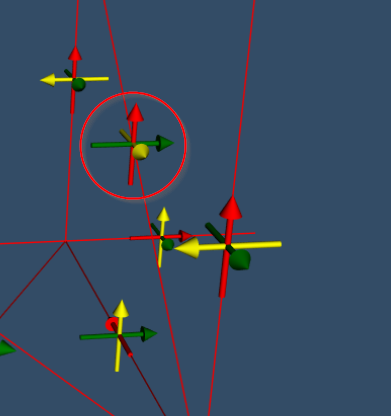# Rotate AxesActor to align axes to three vectors

Hi,

I need to add multiple AxesActors to represent the local coordinate systems of elements. For each element I have a coordinate system which is defined by three direction unit vectors (vx, vy, vz) and an origin point (x, y, z). Set the position of the AxesActor is easy using the setPostionFrom() function and providing the x, y, z tuple.

But how can I rotate the AxesActor so that the x axis is align in direction of vx, y axis in direction of vy and z axis in direction of vz?

I have tried to calculated the angles between the global coordinate system and the three axes and then rotate the AxesActor using rotateX, rotateY and rotateZ. But that isn’t working for all cases.

Thanks!

I have tried to project the x-axis to the plane which is constructed by the global x+y axes and x+z axes. And then calculate the angle of the projected axis the global z- or y-axis:

• vpx = project vx onto plane which is definied by global x and y axis
• calculate angle from vpx to global z-axis
• that should be rotation around the global z-axis

And for the rotation around global y-axis:

• vpx = project vx onto plane which is definied by global x and z axis
• calculate angle from vpx to global y-axis
• that should be rotation around the global y-axis

Rotation around x-Axis = 0.
I then use these three angles to rotate the Coordinate System Actor in vtk-js. That is working in most cases. But it’s for example not working when the local coordinate system is:
XAxis: (0, 0.6401843996644799, -0.7682212795973759), YAxis: (0, 0.7682212795973759, 0.6401843996644799), ZAxis: (1, -0, 0)

Then I need a rotation around the x axis. But If don’t know how and when to calculated this rotation… :-/Any idea how to fix the rotation for all cases?